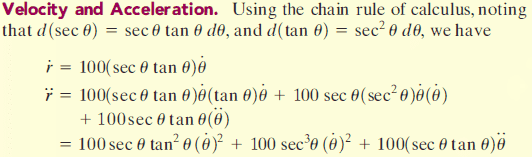# Help deriving an equation using chain rule

## Homework StatementHow does one get the r" equation from r'?

## Homework Equations

r = distance
v = r' = ds/dt
a = r'' = dv/dt

chain rule, dy/dt = dy/dx * dx/dt

## The Attempt at a Solution

I can easily get to r' from r using the chain rule but how do you derive r" from r'? How do you apply the chain rule when there are 3 variables (sec theta, tan theta and theta dot)?

Help would be appreciated.

$\frac{d}{dt} f(a(t),b(t),c(t))=\frac{\partial f}{\partial a}\dot a+\frac{\partial f}{\partial b} \dot b+\frac{\partial f}{\partial c} \dot c$
•